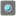• # Code too slow - #contains code#

## Question related to missionPearls in the Box

I need help because my code is too slow to solve the really big cases. Any advice? Thanks

def checkio(marbles: str, step: int) -> float:

```mCount = [marbles.count('w'), marbles.count('b')]

def marbleCount(m, step):

m2 = []
for i in range(0, len(m), 2):
m2 += [m[i] - 1, m[i+1] + 1] * m[i]
m2 += [m[i] + 1, m[i+1] - 1] * m[i+1]
step -= 1
if step > 1:
return marbleCount(m2, step)

else:
return m2

myList = marbleCount(mCount, step)

bCount = sum([myList[i] for i in range(1, len(myList), 2)])
wCount = sum([myList[i] for i in range(0, len(myList), 2)])

return round(wCount / (wCount +bCount),2)
```18